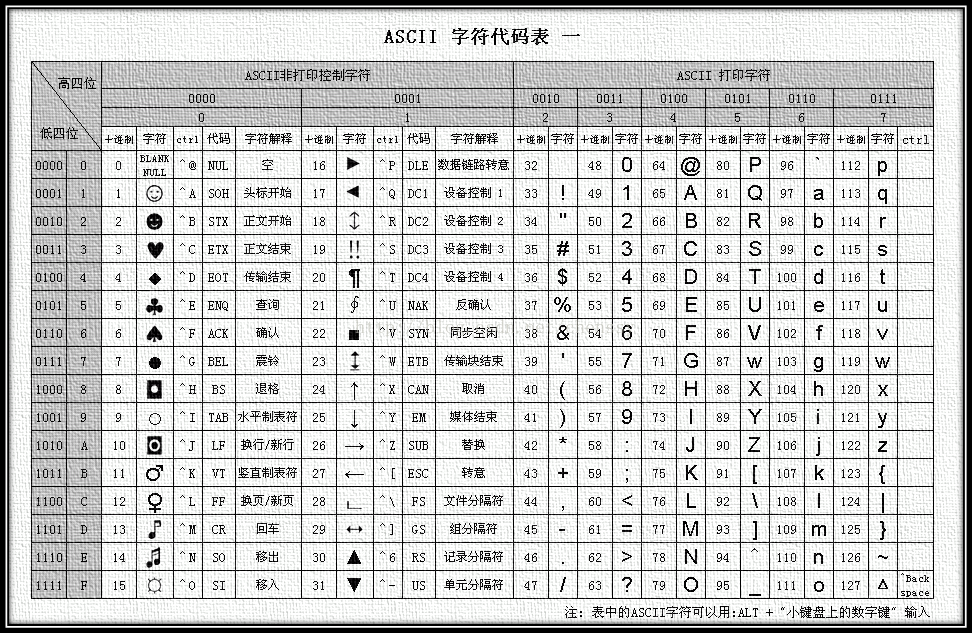• 描述

• 注意:
假设字符串的长度不会超过 1010。

• 示例 1:

"abccccdd"

7

• Solutionans += i % 2 == 0 ? i : --i;

class Solution {
public int longestPalindrome(String s) {
int[] cnt = new int;
for(char c : s.toCharArray()){
cnt[c - 'A'] += 1;
}
int ans = 0;
for(int i : cnt){
ans += i % 2 == 0 ? i : --i;
}
return ans < s.length() ? ++ans : ans;
}
}